理论分析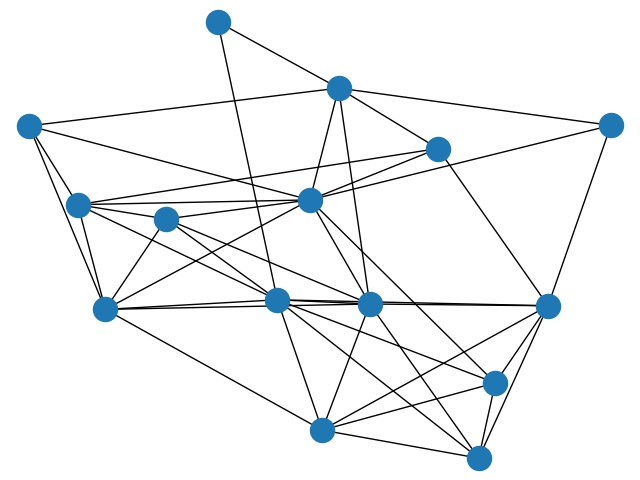社会网络模拟

调整型网络

网络模型

• 生成 $n$ 个节点.
• 按指定的规律, 让每个节点和一部分节点连上边 (即每个人和一部分人成为朋友). 第 $i$ 个节点和第 $i+1,i+2,\cdots, i+r$个节点连边, 其中加法都在模 $n$ 的意义下进行.
• 在总共 $n\cdot r$ 条边随机选择占比为 $p$ 的边删除, 并将每条删掉的边, 随机选则新的边替代. 最终总边数仍为 $n\cdot r$.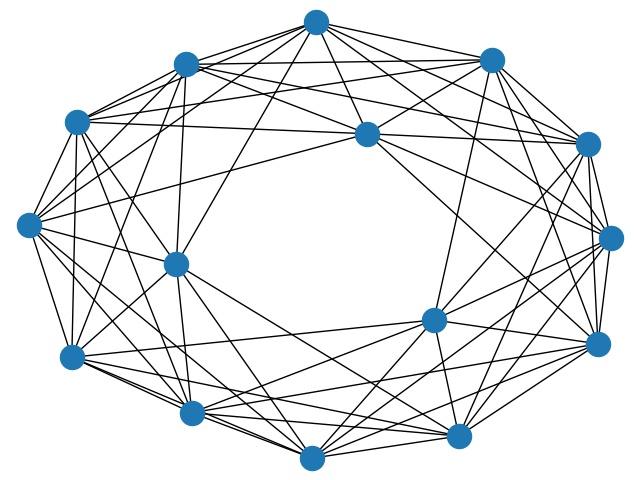实验结果

总人数 $n$ 的实验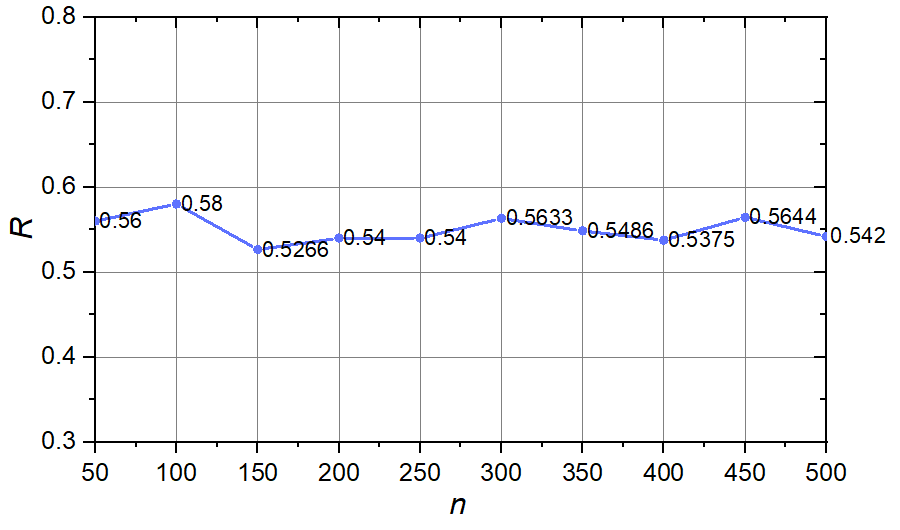初始朋友数 $r$ 的实验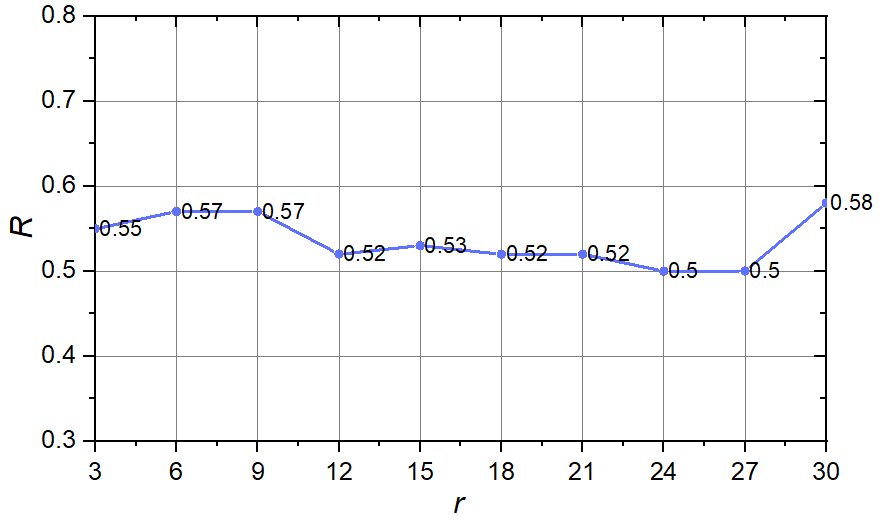调整占比 $p$ 的实验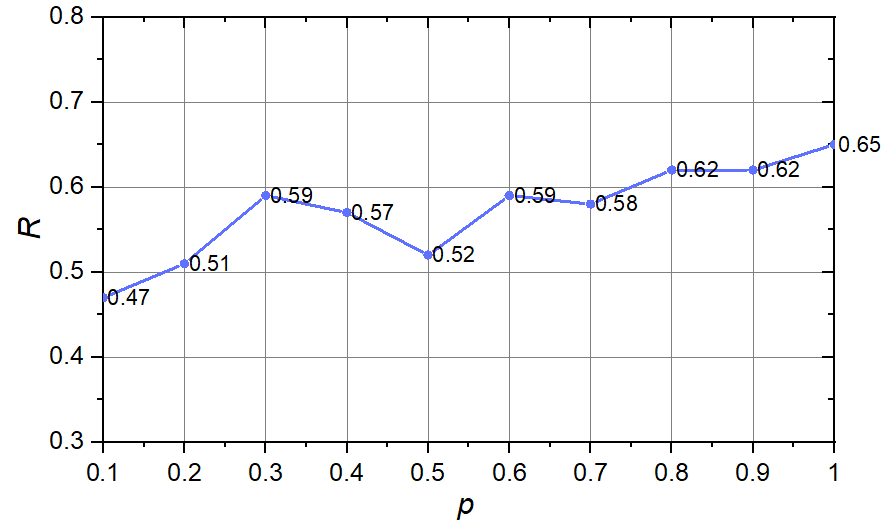随机型网络

网络模型

• 生成 $n$ 个节点.
• 每个节点和随机选择 $r$ 个节点 ( $r$ 是固定的), 并与之连上边 (即每个人和随机和一定数量的人成为朋友).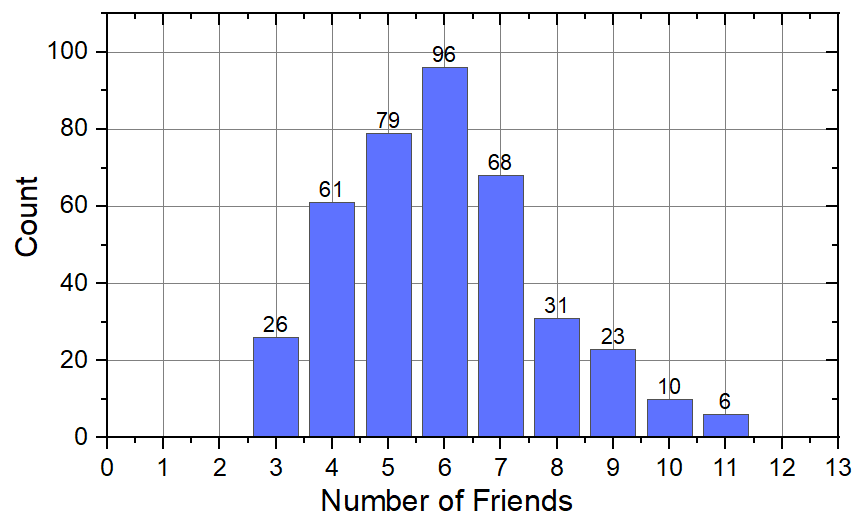实验结果

总人数 $n$ 的实验初始朋友数 $r$ 的实验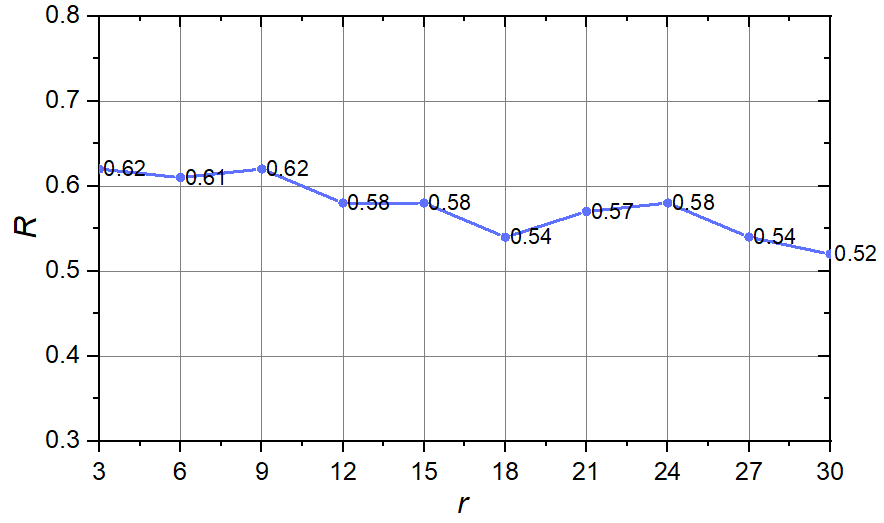总结与思考

• 在随机型网络的基础上加入更多参数, 如每个人的主动交友数量的概率分布;

• 随机生成特定的网络后, 利用一系列增强手段添加或减少边 (甚至可以考虑图神经网络来完成增强), 而非只是随机删减边;

• 采用全新的网络生成思路, 如为每个节点构造各个属性值 (兴趣爱好, 居住地, 教育背景等), 使用聚类的方法生成网络.

Welcome to my other publishing channels